# Correlations of zeros

The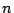-correlation functions of the zeros of the Riemann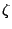-function have been determined for a restricted class of test functions. See [49 #2590][ MR 96d:11093][ MR 97f:11074]. These results are established by relating the correlation functions to a sum over the prime numbers, and at present it is possible to prove a rigorous result only in the range where the diagonal terms'' in the sum are dominant.

Extending to a larger range would require some sort of information on sums of the form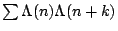, where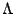is the Von Mangoldt function defined by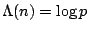if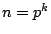,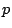prime, and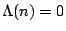otherwise. These sums appear to be closely related to the twin prime'' problem, because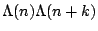is nonzero only whenand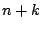are both primes (or prime powers, which is not a significant contribution). Bogolmony and Keating see [Nonlinearity 8, 1115-1131] and [Nonlinearity 9, 911-935], derive all-correlation functions by assuming the Hardy-Littlewood conjectures and ignoring error terms, and the result agrees with the GUE conjecture. The calculation involves some difficult combinatorics.

The Hardy-Littlewood twin prime conjectures are too strong of an input into this problem, because it is averages of sums of the formwhich need to be evaluated, and the information about primes of a specific form is lost in the averaging. In particular, the GUE hypothesis does not imply the Hardy-Littlewood conjectures. Goldston, Gonek, and Montgomery have shown that the pair correlation conjecture is equivalent to a statement about the variation of the distribution of primes. This is not even strong enough to imply that there is a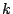for which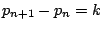infinitely often. It appears that GUE hypothesis for-correlation is equivalent to a statement about the variation in the distribution of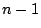-almost primes.

It would be a significant accomplishment to prove anything about the correlation functions outside the range in which they currently are known. Two results in this direction are Özlük's work [ MR 92j:11091] on the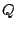aspect of pair correlation for Dirichlet L-functions, and recent work of Goldston, Gonek, Özlük and Snyder [ MR 2000k:11100] in which they prove a lower bound for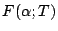for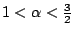.

It also would be valuable to have an idea, assuming GUE, of the rate at which the-correlation sums converge to their limiting behavior, and to have an idea of how that rate changes as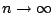. See ratios of zeta-functions for some additional discussion.

Back to the main index for L-functions and Random Matrix Theory.